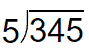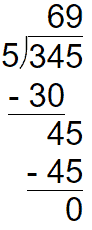# 3-digit by 1-digit Division Worksheets

## All About These 15 Worksheets

These 3-digit by 1-digit Division worksheets are designed to help students master the concept of dividing three-digit numbers by one-digit numbers. The worksheets include a variety of creative and engaging exercises to help students build their skills and confidence in division.

Each worksheet in the series includes a mix of problems with varying degrees of difficulty, designed to challenge and engage students at different skill levels. The problems require students to divide three-digit numbers by one-digit numbers, or find the missing divisor or dividend instead of the quotient in these equations.

This series is most suitable for students in grades 4 to 6. It can be used in the classroom as a supplement to the math curriculum or at home as a way for parents to help their children practice and reinforce their math skills.

Overall, these 3-digit by 1-digit Division workshees provide a comprehensive and engaging way for students to develop their skills and confidence in dividing three-digit numbers by one-digit numbers. With these worksheets, students can build a solid foundation in math and develop the skills they need to succeed in higher-level math courses.

## How to Perform This Math Skill

Dividing a 3-digit number by a 1-digit number is a straightforward process when using long division. Here’s a step-by-step guide that teachers can share with their students:

• Write the Problem – Write the 3-digit number (the dividend) under a long division symbol and the 1-digit number (the divisor) outside the symbol, to the left of the dividend.
• Divide the First Digit – Check if the divisor can be divided into the first digit of the dividend. If it can, write the result (quotient) above the first digit. If it cannot, move to step 3.
• Divide the First Two Digits – If the divisor cannot be divided into the first digit, check if it can be divided into the first two digits of the dividend. Write the result above the second digit.
• Multiply and Subtract – Multiply the divisor by the first digit of the quotient and write the product below the dividend, aligned with the first two digits. Subtract this product from the first two digits of the dividend, and write the difference below.
• Bring Down the Next Digit – Bring down the third digit of the dividend and write it to the right of the difference from the previous step.
• Divide Again – Divide the divisor into the new number you created in step 5, and write the result above the third digit of the dividend.
• Multiply and Subtract Again – Multiply the divisor by the second digit of the quotient and write the product below the new number from step 5. Subtract this product, and write the difference below.
• Check for a Remainder – If the difference in step 7 is zero, then the division is complete. If there’s a non-zero difference, this is the remainder. Write the remainder next to the quotient with an “R” or as a fraction (remainder over divisor).

Example: Let’s divide 345 by 5.

Step 1: Setup Problem

345 is the dividend (gets set within the bracket). 5 is the divisor (gets set outside the bracket).Step 2: Divide into the first digit (Left)

In this case 5 cannot be divided into 3. We will therefore divide into the first two digits. 5 will be divided into 34. This gives us 6 (the start of our quotient), we place that up top and drop the remainder (4).Step 3: We then divide 5 into the entire remainder (45)

45/5 = 9.

The quotient is therefore 69.